Space and PhysicsPUBLISHED

# Two High School Students Discover "Impossible" Proof Of The Pythagorean Theorem

## It's got mathematicians excited for quite a few reasons.8Comments1.1kSharesBuckle in everyone, it's time to do some math. Image credit: Lolostock/Shutterstock

When a result has been around for as long as the one we now know as the Pythagorean Theorem – it’s maybe 4,000 years old at least, at last count – you might expect there wouldn’t be much new to say about it. But as two high school students from New Orleans may now have shown, there’s always more to learn – and in this case, it’s something most mathematicians had written off as impossible for centuries.

“In the 2,000 years since trigonometry was discovered it's always been assumed that any alleged proof of Pythagoras’s Theorem based on trigonometry must be circular,” begins the abstract of the talk given by Ne’Kiya Jackson and Calcea Rujean Johnson at the American Mathematical Society Spring Southeastern Sectional Meeting on March 18, 2023.

“But that isn’t quite true,” they argue. “In our lecture, we present a new proof of Pythagoras’s Theorem which is based on a fundamental result in trigonometry – the Law of Sines – and we show that the proof is independent of the Pythagorean trig identity sin2x + cos2x = 1.”

It’s a result that has caused quite a stir in the mathematical community. “I for one am so excited that two high schoolers were able to come up with this,” says YouTuber MathTrain in a recent video about the proof. “New ideas are hard to come by in a field as well-traveled as this theorem, so I’m excited to see the full paper when it’s released.”

So, what exactly is it that’s getting mathematicians so excited about this new proof? First, let’s remind ourselves of what exactly the Pythagorean Theorem says: given a right-angled triangle, with two sides labeled a and b and the longest side labeled c, the Theorem tells us that

a2 + b2 = c2.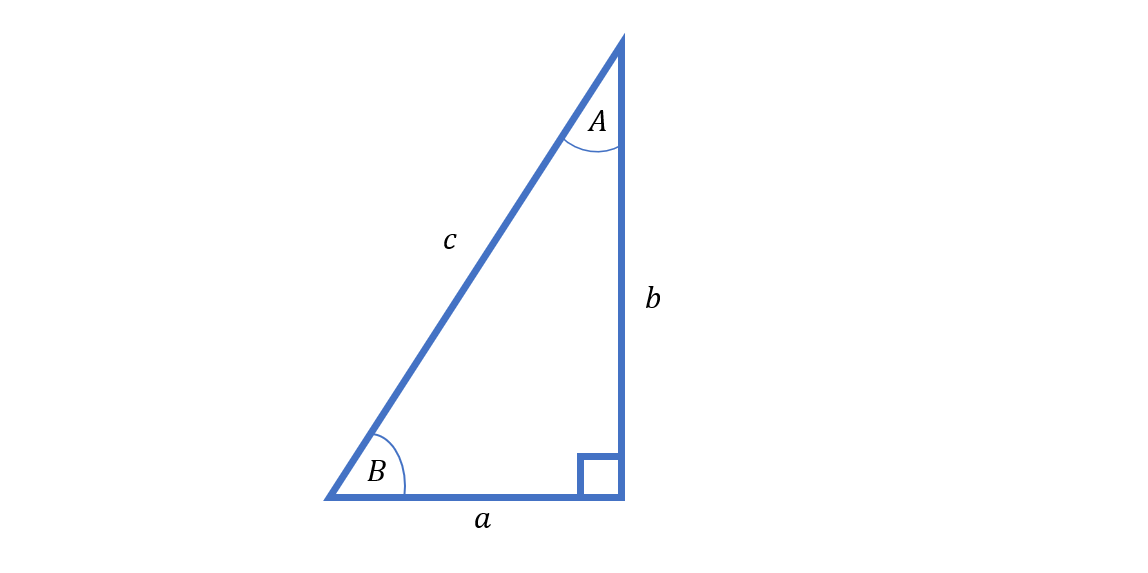There have been literally hundreds of proofs of this little fact presented over the centuries, ranging from simple geometric demonstrations to calculations using differentials or complex numbers.

Very few of those proofs, however, rely on trigonometry – aka the study of angles and ratios of lengths in geometric figures. There’s a good reason for that: most fundamental rules of trigonometry are themselves based on the Pythagorean theorem, meaning any such proof would likely end up begging the question rather than actually making any logical sense.

But as Johnson and Jackson point out, there are some exceptions to that rule – including the result that mathematicians know as the Law of Sines. If we label the angle opposite side a as angle A, the angle opposite side b as angle B, and the angle opposite side c as angle C, then the Law of Sines says that

sinA/a = sinB/b = sinC/c.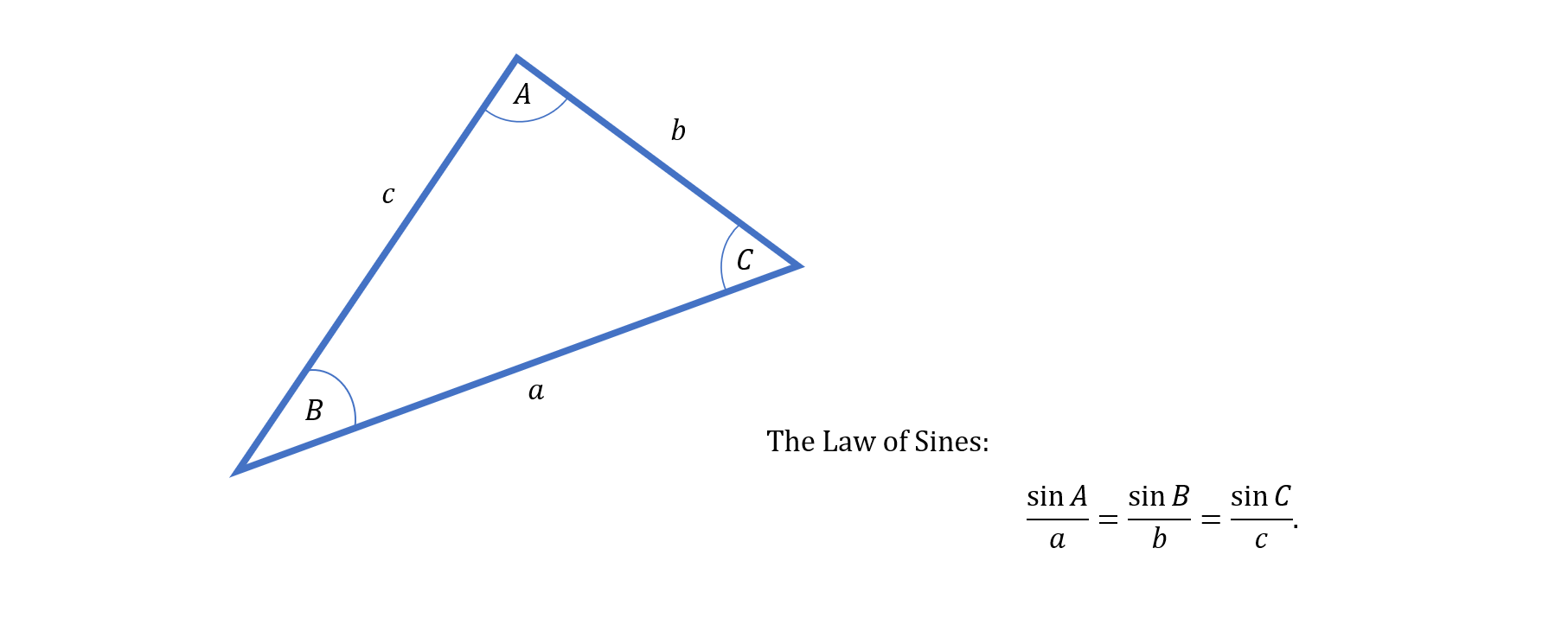Despite being quintessentially a trigonometrical relationship – the sine function is defined as the ratio between two sides of a right-angled triangle, after all – you don’t need anything more advanced than basic geometry to prove this equation. That means it is true independently of the Pythagorean Theorem – and it can therefore be used as the basis for proof without causing any logical headaches.

So far, so good, but how exactly did the pair of budding mathematicians proceed from here? Unfortunately, since the proof was delivered as a presentation rather than a paper, it’s not been published – but luckily for us, a local news station reporting on the girls’ achievement showed some of their slides, and eager online mathematicians have already jumped at the chance to figure out the potential new proof for themselves.

“Zooming in on the slides that you can see as part of the video, you can actually reconstruct the proof fairly easily,” explains MathTrain. “It only took me about an hour… it’s pretty fun to do.”

The proof MathTrain goes on to demonstrate is as simple as it is beautiful. First, we construct a right-angled triangle – probably the first step in any proof of the Pythagorean Theorem – and label the sides and angles. So far, so standard.

The next step is where things get more interesting. We add more triangles to the diagram: first, we double up the original triangle by adding its mirror image to one side; then we extend the hypotenuse of this mirror triangle until it connects with a line perpendicular to the original hypotenuse.

The result is a new right-angled triangle – one with side lengths c, x, and hypotenuse z. But the key to the proof lies not in this bigger figure, but in the smaller ones that fill it up.

“The last part of the construction is to subdivide this big triangle into infinitely many smaller right triangles with are all similar to the original that we drew,” explains MathTrain. “Johnson and Jackson note that the ratio between successive triangles as you go further down this infinite descent is a/b for each triangle.”

From here, we can see that the lengths x and z are simply the sums of the hypotenuses of these successive triangles. And that would be great – except that, of course, there are infinitely many of them to add up.

And so we jump to the world of infinite series – in this case, infinite geometric series. Luckily, these are well-studied and pretty easy to tackle, and a simple formula tells us the lengths we need: we find that

x = c/b2-a2 ∙ 2ab

and

z = c/b2-a2 ∙ (a2 + b2).

It’s easy to see that these equations are pretty similar to each other – “so similar, in fact, that if we take their quotient, these two factors will cancel out, and we’ll be left with just 2ab/a2 + b2,” MathTrain points out.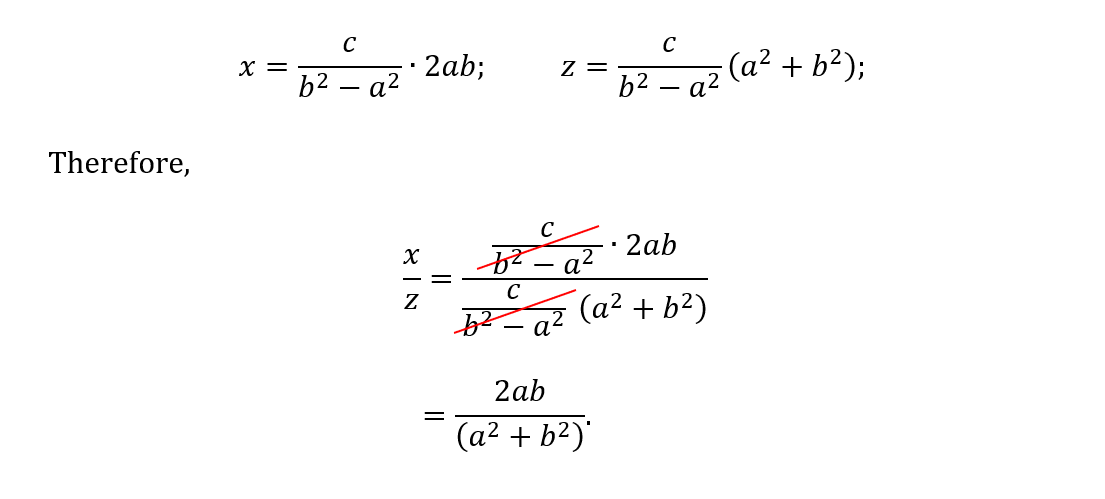And now, finally, the Law of Sines comes into play. By definition of the sin function, we know that x/z is equal to sin(2A) – and by applying the Law of Sines to the triangle created by our original right-angled triangle plus its mirror image, we can see that sin(2A) is in turn equal to 2ab/c2.

This leaves us with something which is already looking suspiciously like the Pythagorean Theorem: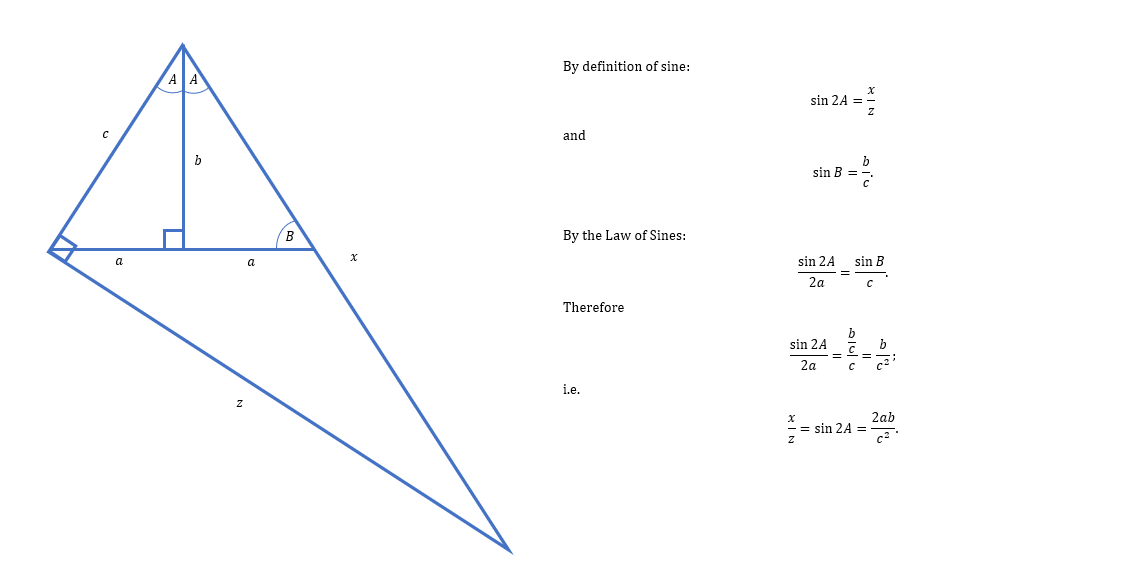And indeed, just a little rearranging and cancelation reveals the equation was there all along.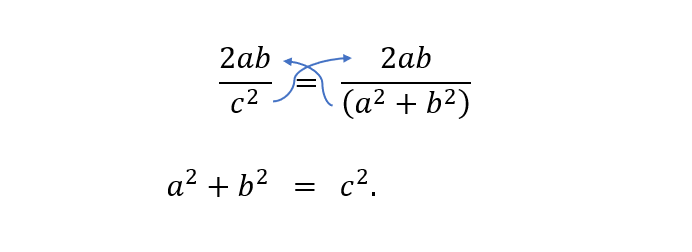“While I’m not sure if this is exactly how Johnson and Jackson did the proof, [this] one… should be pretty similar based on the news reports that I saw,” MathTrain says. “The only major theorems I saw this relying on were the angle-angle theorem and the Law of Sines, both of which have proofs which are completely independent of the Pythagorean Theorem.”

Of course, like any mathematical argument, the proof will need to be peer-reviewed before it is formally accepted – but the math, at least, seems watertight. The pair have been encouraged by the American Mathematical Society to send their result for just such review, with Catherine Roberts, executive director for the AMS, telling The Guardian that it would allow “members of our community [to] examine their results [and] determine whether their proof is a correct contribution to the mathematics literature.”

“[The AMS] celebrate[s] these early career mathematicians for sharing their work with the wider mathematics community,” Roberts added. “We encourage them to continue their studies in mathematics.”

As for Johnson and Jackson, the pair are rightfully elated by their achievement. “There’s nothing like it – being able to do something that people don’t think that young people can do,” Johnson told local New Orleans news station WWLSpace and Physics
•